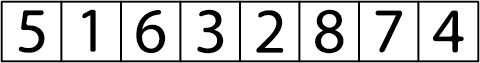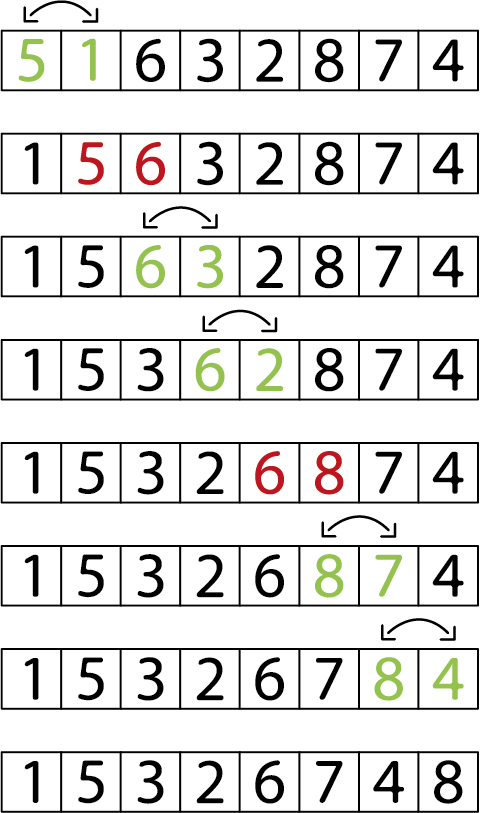# Bubble Sort in Javascript: A Step-By-Step Tutorial

Here we go for a series of articles about sorting algorithms.
For this first part, we will review the Bubble sort algorithm, or Bubble Sort in English.

## How Bubble Sort works

The principle is simple and the name Bubble sort is not chosen by chance :

• The largest values considered as heavy sink progressively to the bottom of the list.
• The smallest values considered as light rise like bubbles to the top of the list.

Let's take the following number series :By iterating over each number in the series, if the current number is greater than the next number, then we swap their positions :When a complete loop over the series is done without any swap being performed, the sorting operation is done.## Code example

Let's translate all that into code with a Javascript example :

``````const swap = ( arr, i, j ) => {
let temp = arr[ i ];
arr[ i ] = arr[ j ];
arr[ j ] = temp;
}

const bubbleSort = arr => {
for ( let i = 0; i < arr.length - 1; i++ ) {
let swapped = false;
for ( let j = 0; j < arr.length - 1; j++ ) {
if ( arr[ j ] > arr[ j + 1 ] ) {
swap( arr, j, j + 1 );
swapped = true;
}
}
// SI aucun nombre n'a été permuté lors de la boucle précedente
// ALORS le tri est terminé et on peut sortir de la boucle
if ( !swapped ) {
break;
}
}

return arr;
}

const arr = [ 2, 1, 4, 3, 5, 7, 6, 8 ];
bubbleSort( arr ) // [ 1, 2, 3, 4, 5, 6, 7, 8 ]``````

## Performances

Do not use this algorithm in production! Although it is easy to understand, bubble Sort is actually much less performant than other of its peers, such as Quick Sort or Insertion Sort that we will study in future articles.
Bubble Sort is mainly presented as an educational tool to introduce beginners to sorting algorithms.